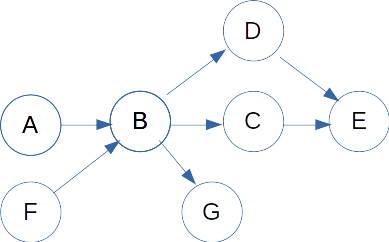## dimanche 26 septembre 2021

### Order of GeoJSON properties and graph theory

All this story started with this QGIS bug about a crash when a user adds a new field to a GeoJSON file. Who is the culprit between GDAL and QGIS could be debated. Let's look at the GDAL side of things.

Let's consider this simple GeoJSON file:

{ "type": "FeatureCollection", "features": [
{ "type": "Feature", "geometry": null, "properties": { "A": "a", "C": "c" },
{ "type": "Feature", "geometry": null, "properties": { "A": "a", "B": "b", "C": "c" }
]}

GDAL versions up to 3.3.x will expose a layer definition with the fields in the following order, [A, C, B], and this is what confused QGIS, since the C field was appended as the last action, and QGIS expected it to be the last field.

One could also argue that we should not worry about the order of properties since a JSON object such as the "properties" one is unordered. However the JSON parser and writer used by GDAL has the property of respecting the order of appearance of the keys which makes GeoJSON a format that behaves similarly to other formats such as Shapefile, GeoPackage or CSV where the field order is fixed. But in a GeoJSON file where there is no declaration of properties (no "header"), and when properties are omitted in some features, presenting a consistent field order is  more challenging.

How does current GDAL in current proceeds to build the list of fields ? That's very simple: iterate over features, for each feature, iterate over its properties, and as soon as a new property is found, append it to the list of properties of the layers. So on our above example, after the first feature, we have [ A, C ]. And when parsing the second feature, we append B to it, hence [A, C, B ]. We can also notice that the order in which features appear in the collections influences the order of properties exposed in the layer definition.

How to fix that ? For that very simple case, we could say "B appears after A, which is already in our list, and before C, which is already in our list and was just after A, so let's insert it in between". Great ! Problem solved ? Unfortunately not.

What if you see features with the following properties:

- [A, D], ==> current consolitated list [A, D]

- then [A, B, D], ==> current consolitated list [A, B, D]

- then [C, D], ==> hum C is before D, but where relatively to A and B ??

- then [A, C], ==> we know now that C is after A. But before or after B ?

- then [B, C], ==> ok, before B. So final list is [A, B, C, D]

Our simple logic of insertion no longer works here, and we can know feel that the problem is much more involved than what it initially looked like. We could also have situations where the dataset is so sparse that the relative order of some fields is indeterminate. Or where fields appear in non consistent order in several features.

My initial though was to use a sorting algorithm, such as std::sort in the standard C++ library, with a custom comparison function that would use the (i, j) apparition order ( (i, j) means that field i immediately appears before j in a feature ). But there are several issues:

- the sort algorithm may invoke the comparison function with any pair of field names, but we have only a knowledge of a subset of those. If we build a graph where the nodes are the field names and a directed edge between i and j means that field i immediately appears before j in a feature, then we can know if there's a path between any tuple of nodes by using standard graph algorithm.

- the comparison function of std::sort implies that we have a strict weak ordering. When there's a path in the graph between 2 nodes, the ordering is clear. We note immediately that the graph should not have cycles, otherwise we would violate the asymmetry property of the strict weak ordering. So when building the graph, we should reject any new edge i->j that would result in a cycle, which boils down to finding if the reverse path j->i already exists. But what to do for nodes that aren't related by a path ? We could decide that they are incomparable. However the "transitivity of incomparibility" property could then be violated. For example if there's no path between A and B, and between B and C (for example B is an isolated node), there might be a path between A and C. So it is far from obvious that we can define a comparison function that respects the strict weak ordering. And if it failed to respect it, then the std::sort() execution is undefined behaviour, meaning that anything can happen: at best, a wrong result; at worse, a crash, infinite loop, etc. Look at this post for other examples of comparision function that subtely violate strict weak ordering

At the end, we want to somewhat "linearize" our graph, by respecting the directed edge.

So given:We want to get a rresult like [A, F, B, G, D, C, E], or [F, A, B, C, D, E, G], or ...

It appears that this is a known problem in graph theory: computing the topological ordering of a directed acyclic graph. As show in the above example, there are in general several solutions. To try to make the result less "random", we use lexicographical order of field names for the working set S of the Kahn's algorithm, which is an additional sorting rules for nodes that have no direct relationship in the graph.

The result of that work is in https://github.com/OSGeo/gdal/pull/4552